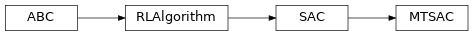# garage.torch.algos.mtsac¶

This modules creates a MTSAC model in PyTorch.

class MTSAC(policy, qf1, qf2, replay_buffer, env_spec, *, num_tasks, eval_env, gradient_steps_per_itr, max_episode_length_eval=None, fixed_alpha=None, target_entropy=None, initial_log_entropy=0.0, discount=0.99, buffer_batch_size=64, min_buffer_size=int(10000.0), target_update_tau=0.005, policy_lr=0.0003, qf_lr=0.0003, reward_scale=1.0, optimizer=torch.optim.Adam, steps_per_epoch=1, num_evaluation_episodes=5, use_deterministic_evaluation=True)A MTSAC Model in Torch.

This MTSAC implementation uses is the same as SAC except for a small change called “disentangled alphas”. Alpha is the entropy coefficient that is used to control exploration of the agent/policy. Disentangling alphas refers to having a separate alpha coefficients for every task learned by the policy. The alphas are accessed by using a the one-hot encoding of an id that is assigned to each task.

Parameters
• policy (garage.torch.policy.Policy) – Policy/Actor/Agent that is being optimized by SAC.

• qf1 (garage.torch.q_function.ContinuousMLPQFunction) – QFunction/Critic used for actor/policy optimization. See Soft Actor-Critic and Applications.

• qf2 (garage.torch.q_function.ContinuousMLPQFunction) – QFunction/Critic used for actor/policy optimization. See Soft Actor-Critic and Applications.

• replay_buffer (ReplayBuffer) – Stores transitions that are previously collected by the sampler.

• env_spec (EnvSpec) – The env_spec attribute of the environment that the agent is being trained in.

• max_episode_length_eval (int or None) – Maximum length of episodes used for off-policy evaluation. If None, defaults to max_episode_length.

• eval_env (Environment) – The environment used for collecting evaluation episodes.

• gradient_steps_per_itr (int) – Number of optimization steps that should occur before the training step is over and a new batch of transitions is collected by the sampler.

• fixed_alpha (float) – The entropy/temperature to be used if temperature is not supposed to be learned.

• target_entropy (float) – target entropy to be used during entropy/temperature optimization. If None, the default heuristic from Soft Actor-Critic Algorithms and Applications is used.

• initial_log_entropy (float) – initial entropy/temperature coefficient to be used if a fixed_alpha is not being used (fixed_alpha=None), and the entropy/temperature coefficient is being learned.

• discount (float) – The discount factor to be used during sampling and critic/q_function optimization.

• buffer_batch_size (int) – The number of transitions sampled from the replay buffer that are used during a single optimization step.

• min_buffer_size (int) – The minimum number of transitions that need to be in the replay buffer before training can begin.

• target_update_tau (float) – A coefficient that controls the rate at which the target q_functions update over optimization iterations.

• policy_lr (float) – Learning rate for policy optimizers.

• qf_lr (float) – Learning rate for q_function optimizers.

• reward_scale (float) – Reward multiplier. Changing this hyperparameter changes the effect that the reward from a transition will have during optimization.

• optimizer (torch.optim.Optimizer) – Optimizer to be used for policy/actor, q_functions/critics, and temperature/entropy optimizations.

• steps_per_epoch (int) – Number of train_once calls per epoch.

• num_evaluation_episodes (int) – The number of evaluation episodes used for computing eval stats at the end of every epoch.

• use_deterministic_evaluation (bool) – True if the trained policy should be evaluated deterministically.

to(self, device=None)

Put all the networks within the model on device.

Parameters

device (str) – ID of GPU or CPU.

train(self, trainer)

Obtain samplers and start actual training for each epoch.

Parameters

trainer (Trainer) – Gives the algorithm the access to :method:~Trainer.step_epochs(), which provides services such as snapshotting and sampler control.

Returns

The average return in last epoch cycle.

Return type

float

train_once(self, itr=None, paths=None)

Complete 1 training iteration of SAC.

Parameters
• itr (int) – Iteration number. This argument is deprecated.

• paths (list[dict]) – A list of collected paths. This argument is deprecated.

Returns

loss from actor/policy network after optimization. torch.Tensor: loss from 1st q-function after optimization. torch.Tensor: loss from 2nd q-function after optimization.

Return type

torch.Tensor

optimize_policy(self, samples_data)

Optimize the policy q_functions, and temperature coefficient.

Parameters

samples_data (dict) – Transitions(S,A,R,S’) that are sampled from the replay buffer. It should have the keys ‘observation’, ‘action’, ‘reward’, ‘terminal’, and ‘next_observations’.

Note

samples_data’s entries should be torch.Tensor’s with the following shapes:

observation: $$(N, O^*)$$ action: $$(N, A^*)$$ reward: $$(N, 1)$$ terminal: $$(N, 1)$$ next_observation: $$(N, O^*)$$

Returns

loss from actor/policy network after optimization. torch.Tensor: loss from 1st q-function after optimization. torch.Tensor: loss from 2nd q-function after optimization.

Return type

torch.Tensor

property networks(self)

Return all the networks within the model.

Returns

A list of networks.

Return type

list